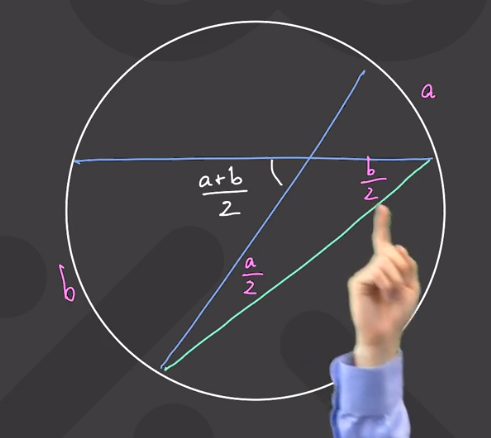# So for any a and b, the angle of the intersection of the chords will always be a+b/2 no matter what size a and b are and no matter where the intersection is?

• This post is deleted!

• [Originally posted in the Discussions]

So for any a and b, the angle of the intersection of the chords will always be a+b/2 no matter what size a and b are and no matter where the intersection is?

• Yes, that's right! It's very good to be thinking, "But does this only work for this diagram? What if we were to draw the intersecting chords differently?" That's why Prof. Loh tries to prove or illustrate why every theorem is true, so you can see when it can be applied. The proof in this case can be seen in the diagram from the video:You can see that the angle that is formed from the intersection of the chords is equal to

$$\frac{a + b}{2}$$

This is true because this angle is the exterior angle of the triangle containing the green line. Use the fact that the exterior angle of a triangle is equal to the sum of the other two angles of the triangle.

The great thing about trying to prove why identities and theorem are true is that it helps you remember them, also. And it helps you to analyze new situations with a clever, creative approach. Great job on thinking about this idea!

Happy Learning,

The Daily Challenge Team

• This post is deleted!